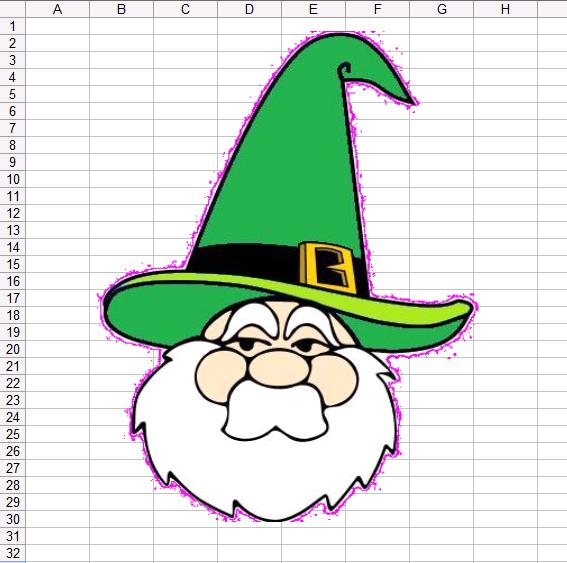# How to Use the Vlookup Function on Excel?

Posted by Lior Weinstein on Thursday, Mar 6th, 2014
Category : Microsoft ExcelExcel is one of the most used software programs for both for business and household purposes Thanks to the fairly intuitive spreadsheets, Excel can be used to document all sorts of information in a concise and accurate manner. Vlookup, which stands for vertical lookup, is a helpful function that allows you to search your spreadsheet for the information you need. There are a number of different things that Vlookup can accomplish; you just need to know how to use it. Here is a short tutorial that will help you use Vlookup successfully.

## Lookup_Value

Vlookup works by entering various formulas that relate to the information found in the spreadsheet. The first piece of syntax one should know is lookup_value. This refers to the value of the cell you are interested in. For instance, If the value of A6, the desired cell, is 25, then the first bit of information you would include in the formula would be 25, and it would look like this: =VLOOKUP(25). The rest of the formula would follow the number 25.

## Table Array

The next required syntax for Vlookup is called table_array. This refers to the range in the spreadsheet that you will be searching. If you are looking at the entire spreadsheet, which consists of cells A2 to D37, then that is your table_array. This means four columns, A, B, C, and D are being used and 36 rows, from row 2 and on, are being used. The formula should now look like this: =VLOOKUP(25, A2:D37).

## Col Index Num

Finally, col_index_num is used to determine the column of the particular cell you want to pull answers from. If the answers are in column A, you would be looking for column 1. The formula would look like this: =VLOOKUP(25, A2:D37, 1).

## Range Lookup

The next bit of syntax is not required. When you include range_lookup in the formula you are asking to determine whether the information that is being gathered will be accurate or not. If you wish only for accurate results, you would include FALSE in the formula. If you want it to be accurate or closest to it, you would include TRUE to the formula. Bear in mind that if you choose the latter option, the cells must be in ascending order in the column that you are working with. This is not the case for the former option. If your are looking for accurate results the formula will look like this: VLOOKUP(25, A2:D37, 1, FALSE).

## Summary

This is the basic tutorial for using Vlookup. It can get more complex based on what you would like to do. In the example we gave, number 25 was a product ID number correlating with a product name. Based on the formula, the results would show that in column A the product tissues correlated with the value, 25, its product ID number.

## Latest From Our Blog### Calculate Your Retirement with Excel and Beat the Recession### How to become 10X more focused at work### How to Master Word & Excel Keyboard Shortcuts in 30 Days